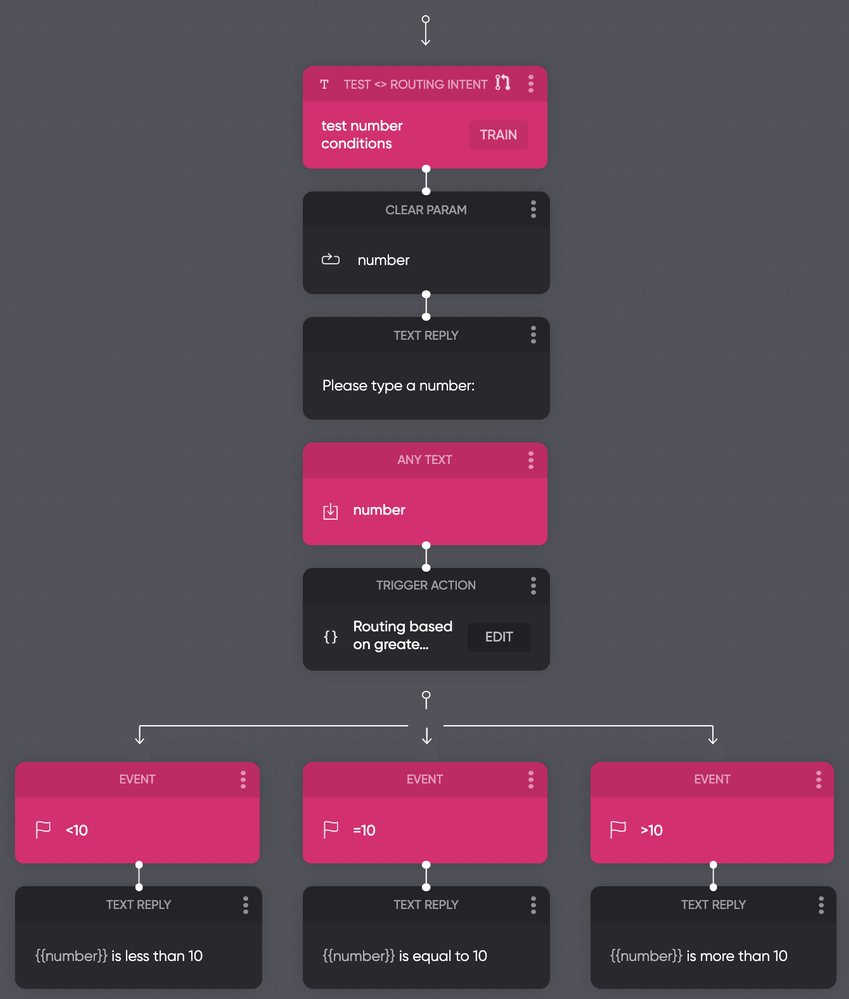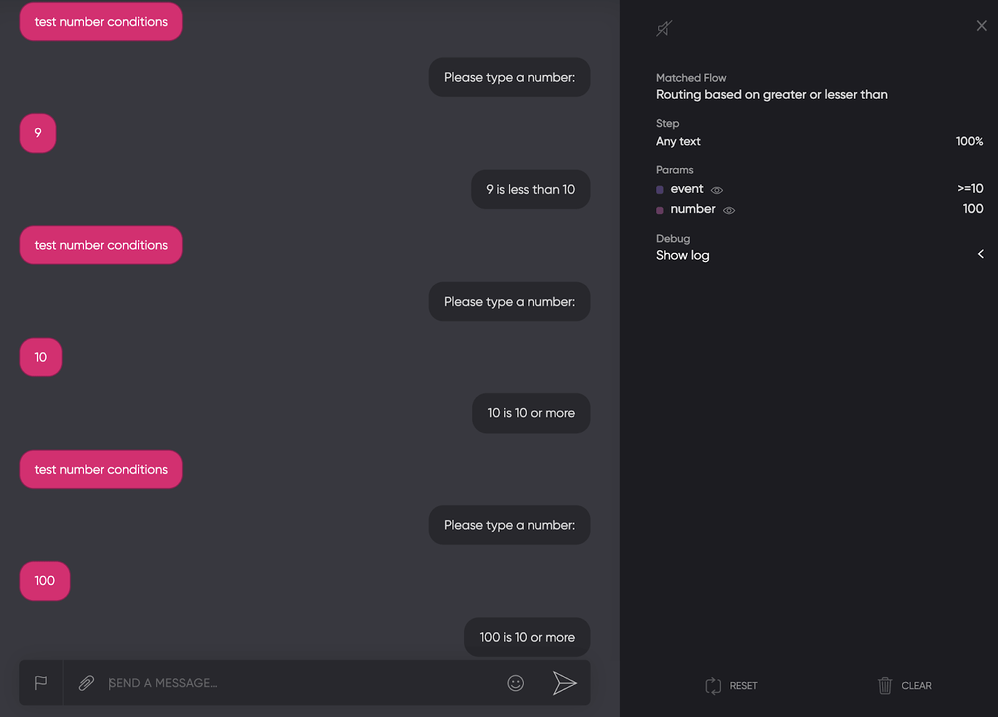# Code-based Actions: Route your bot for a specific number

You can design your flow for the users to type a specific number or a range of numbers for a flow/ event to be triggered.

### Different routing for values that are not less than a specific number

If you want the bot to give a different response when a number is greater, equal, or less than a specific number, you can use a simple code action. For example, you want the bot to check if the number the user enters is either lower than 10, equals 10, or greater than 10.The code action in the flow above contains the following code:
The code action first checks if the number is less than 10, and if so, it will trigger the <10 event. Else, it will trigger the >=10 event.

``````async payload => {

{
trigger('\<10')
}

else
{
trigger('>=10')
}

}
``````

Ensure that you change the event names for your own event names if you copy and paste this code into your own project.

Let's test the above example using flow:### Different routing for values that are equal to a specific number

Let’s see another event for the flow to check if the user input is equal to 10:

### Value is NOT equal to

It also works the other way around. The example above illustrates you can use “payload.params.number.value === 10” to check if the parameter is 10, but you also have the possibility to check if it is not equal to 10.

``````async payload => {

{
trigger('\<10')
}

{
trigger('=10')
}

else
{
trigger('>10')
}

}
``````

To do that, simply replace “===” with “!==”.

### Values in a range

You can also check if the value is in a range (between 2 specific numbers).

To illustrate this, we created another flow:This code action contains the following code:

``````async payload => {